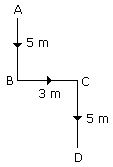﻿

### Reasoning

ISBT Reasoning for all banking PO,Clerk,IBPS PO,Railway,SSC,IAS,OAS Exams

##### Directions : Study the following letter number arrangement and answer the questions given below. B 8 4 C R M 9 N P D K W F A 2 E J 7 X U Q H L T Y 6 G SIn the first half of the above arrangement is written in reverse order followed by the second half written in reverse order, which letter/ number will be eighteenth from left ?
 1) 7 2) Y 3) K 4) C 5) None of these
Explanation : So,  answer should be 'Y'.View Answer
##### Directions : Study the following letter series and answer the questions below. A B C D E F G H I J K L M N O P Q R S T U V W X Y Z Which letter will be seventh to the left of tenth letter from the right-hand side of the above letter series ?
 1) K 2) I 3) J 4) L 5) None of these
Explanation :
If  question is from right to left, it should be added i.e.
7 + 10 = 17 So, seventeenth letter from right will be 'J'.View Answer
##### Directions : Study the following letter series and answer the questions below. A B C D E F G H I J K L M N O P Q R S T U V W X Y Z Which letter will be fourth to the right of eleventh letter from the left-hand side of the above letter series.
 1) O 2) Q 3) L 4) N 5) None of these
Explanation :
If  question is from left to right, it should be added i.e. 11 + 4 = 15 So, fifteenth letter from left will be 'O'.View Answer
##### If the letters of the word CENTRIFUGAL are arranged alphabetically from left to right, which letter will be third to the right of the seventh letter from your right ?
 1) U 2) N 3) C 4) I 5) None of theseView Answer
##### What should come in the place of question mark (?) in the following letter-number series ? M3V O5U R7T T9S W11R ?
 1) Y13Q 2) Z13Q 3) Y15Q 4) Y13P 5) None of theseView Answer
##### What will come in the place of question mark (?)? Z A X C ?
 1) W 2) U 3) E 4) D 5) None of theseView Answer
##### In the following letter series which of the alternatives given below will come in the place of questions mark (?) ? ef vu gh ts ij rq kl po ?
 1) jk 2) lm 3) mn 4) nm 5) None of theseView Answer
##### What will come in place of the question mark (?) in the following letter series ? CDEF DFFG EHHH  ? GLOJ
 1) GJKI 2) FJKI 3) FJJI 4) HJKI 5) None of theseView Answer
##### Vivek started to walk straight towards south. After walking 5 m he turned to the left and walked 3 m. After this he turned to the right and walked 5 m Now to which direction  Vivek is facing ?
 1) South 2) North 3) South-West 4) North-East 5) None of theseView AnswerView Answer